Question 1

# A cube is made by folding the given sheet. In the cube so formed, what would be the symbol on the opposite side of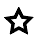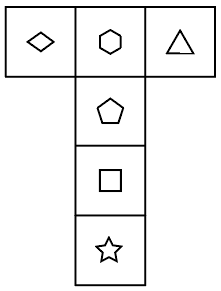Solution

Given figure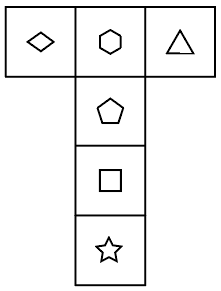from the above figure, the opposite symbol is given below in the figure every third symbol is opposite every first symbol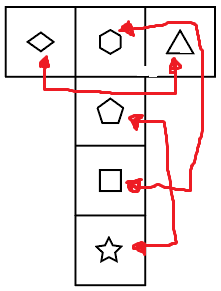then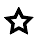opposite symbols are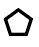therefore Option (A) Ans